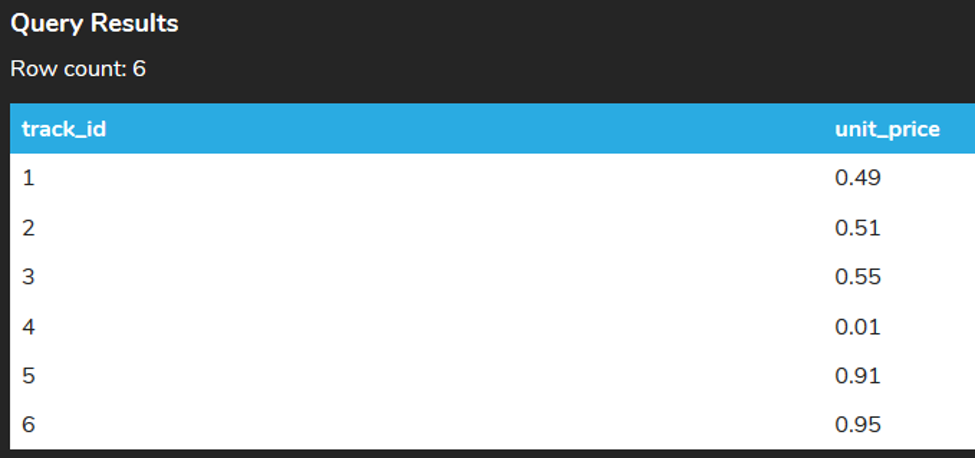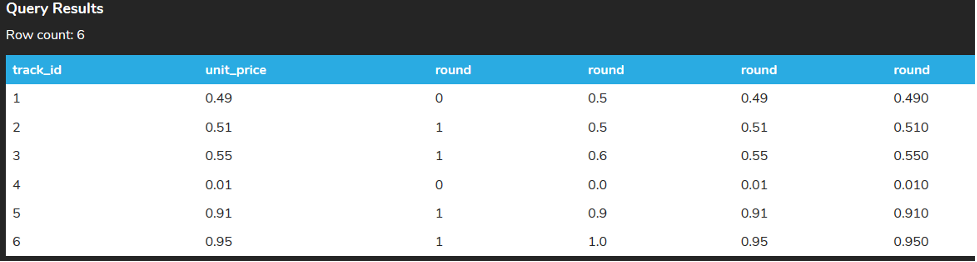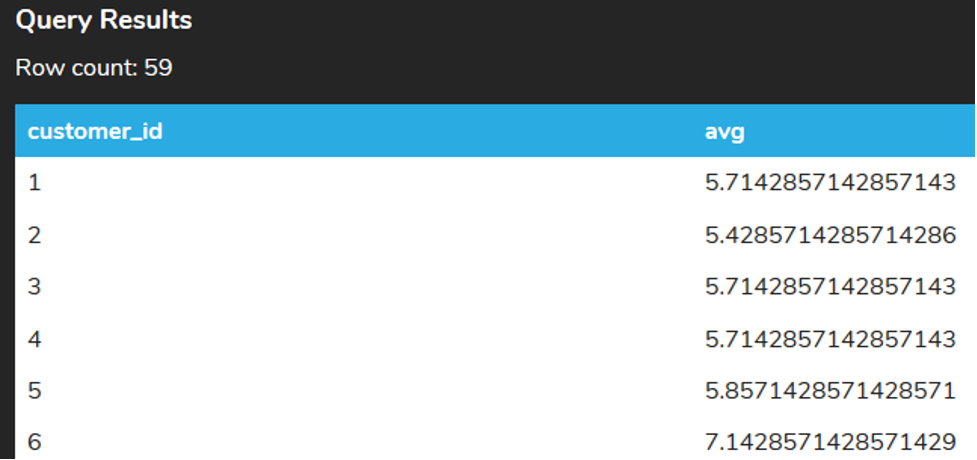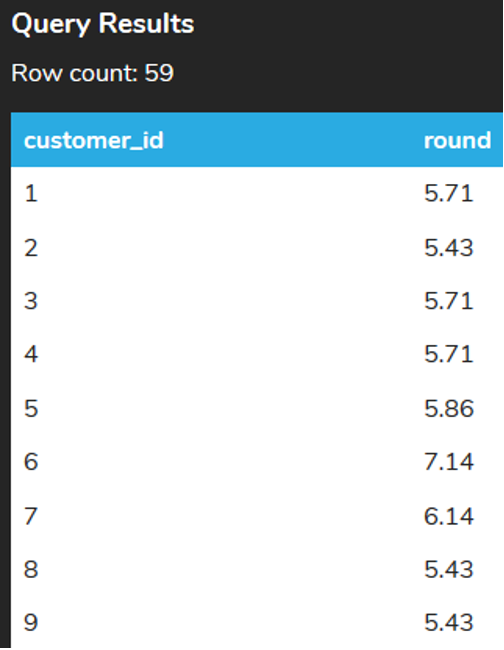+

# ROUND to Round Numbers

##### Rating:
(0)
Author: Sophia Tutorial
##### Description:

Compose a query that utilizes the ROUND function to display numeric values from a given column in a more readable format.

(more)
Tutorial

what's covered
This tutorial explores using the ROUND function to round numbers in three parts:
1. Using the ROUND Function
2. Many Ways To Round
3. Rounding Averages

## 1. Using the ROUND Function

Although we did take a look at the ways to cast values to numeric values, this is a feature that doesn’t exist in all databases. The ROUND function is one that does exist in most databases that rounds a numeric value to the nearest integer or within a number of decimal places.

The ROUND function takes in two arguments. The first is the number, column or expression to be rounded and the second one is the number of decimal places to be rounded to. If you do not include the second argument, it defaults to 0, meaning that it will round to the nearest integer.

Let us run a quick script to make some updates to the track table so that we can take a further look at how the ROUND function works:

``````
UPDATE track SET unit_price = .49 WHERE track_id = 1;
UPDATE track SET unit_price = .51 WHERE track_id = 2;
UPDATE track SET unit_price = .55 WHERE track_id = 3;
UPDATE track SET unit_price = .01 WHERE track_id = 4;
UPDATE track SET unit_price = .91 WHERE track_id = 5;
UPDATE track SET unit_price = .95 WHERE track_id = 6;
``````

We can now take a look at what this would look like ordered based on track_id. The LIMIT clause can be used to only display the first six rows.

``````
SELECT track_id, unit_price
FROM track
ORDER BY track_id
LIMIT 6;
``````## 2. Many Ways To Round

Let’s take a look at what this would look like if we rounded based on various parameters:

``````
SELECT track_id, unit_price, ROUND(unit_price), ROUND(unit_price,1),ROUND(unit_price,2),ROUND(unit_price,3)
FROM track
ORDER BY track_id
LIMIT 6;
``````The first round is based on the closest integer. Any value of 0.50 or higher would be rounded to 1 whereas less than 0.50 would be rounded to 0.

The second round rounds to the closest tenth. Most of this will be obvious but it is important to also look at how the round works in the 6th row to round up to 1.0.

The third round rounds to the nearest hundredth. No changes with the data appear here.

The fourth round is an interesting one as it rounds to the nearest thousandth. Although the data is stored with only two decimal places, as you’ll see, it can add that extra decimal to keep values consistent.

## 3. Rounding Averages

Where the round can be most useful is within calculations like those that use the AVG function, as the data can be a bit complex.

For example, if we want to get the average total of invoices for each customer, the query would look like the following:

``````
SELECT customer_id, AVG(total)
FROM invoice
GROUP BY customer_id
ORDER BY customer_id;
``````

The result would look like the following:Being that the total is based on currency, it makes sense to round it to the nearest cent (two decimal places).

We could do that by adding the ROUND function around the AVG function.

``````
SELECT customer_id, ROUND(AVG(total),2)
FROM invoice
GROUP BY customer_id
ORDER BY customer_id;
``````try it
Your turn! Open the SQL tool by clicking on the LAUNCH DATABASE button below. Then enter in one of the examples above and see how it works. Next, try your own choices for which columns you want the query to provide.

summary
The ROUND function can be used to round numbers, columns and calculations to the nearest decimal or integer.

Source: Authored by Vincent Tran

Rating Header